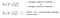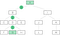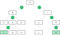# Degrees of separation on a tree algorithm

Imagine that you are like me and looking for an algorithm to compute degrees of separation along a hierarchical tree like the ones pictured below. Your tree could represent any data — let’s pretend it’s a company orgchart with node A as the CEO, nodes B and I are execs, and so on. The distance, or degrees of separation, between any two nodes is the number of links along the shortest path that separates them.

## ConceptX = m’s distance from A, Y = n’s distance from A, Z = number of nodes that m and n share

Say we have selected two nodes m and n, where m = node B and n = node F. Notice that B is F’s eventual manager (via node D). In this case, B’s distance from A, or X, = 1; F’s distance from A, or Y, = 3. The only node B and F have in common is A, so their intersection Z = 1. B and F’s degrees of separation = 2 because 1+3–2(1) = 2.Case 1: One node is the (eventual) manager of the other

Now let’s select two nodes which are not eventual managers of each other — nodes F and H. Both node F’s and H’s distance from A, or X and Y, = 3. Their intersection Z = 3 because they have nodes A, B, and D in common. F and H’s degrees of separation = 2 because 3+3–2(3–1) = 2.

Wait a second, where did that minus 1 come from?! Well, technically it’s minus 2 once you distribute the 2, and it comes from eliminating the double-counting that happens when links are shared by both m and n on their paths to A. I urge you to trace some paths of your own and compute the math to make evident this concept.Case 2: Neither node falls in the management chain of the other

## Code

`degrees <- function(m,n){     ## compute m & n's distance from A     ## subtract 1 to remove "person" column          mFromA <- sum(!is.na(data[m, ])) — 1     nFromA <- sum(!is.na(data[n, ])) — 1     ## compute how many nodes m and n have in common     ## in many cases, m and n only have A in common     overlap <- intersect(data[m, which(!is.na(data[m, ]))], data[n, which(!is.na(data[n, ]))])     ## (optional) print above values      print(paste(“Distance”, data[m,1] ,”to A: “, mFromA))     print(paste(“Distance”, data[n,1] ,”to A: “, nFromA))     print(paste(“length of overlap: “, length(overlap)))     print(overlap)     ## ok, algorithm time:      ## but first, let's check if the user entered the same person      ## i.e. does m == n? if so, degrees of separation = 0     if(m == n){          degreesOfSep <- 0     }      ## check if m or n is (eventual) manager of n or m     ## i.e. check if higher node manages lower node     ## if so, compute distance as X+Y-2*Z     else if(mFromA != nFromA){          if(mFromA > nFromA){ lower <- m; higher <- n }          if(nFromA > mFromA){ lower <- n; higher <- m }          for (i in 2:length(data[lower, ])) {               if(grepl(data[higher,1], data[lower,i])){                    manager <- TRUE                    print(paste(data[higher,1], “is an (eventual) manager of”, data[lower,1]))                    break               }               else{                    manager <- FALSE               }          }          if(manager){               degreesOfSep <- mFromA + nFromA — 2*(length(overlap))          }          ## if one is not (eventual) manager of other,           ## compute as X+Y-2*(Z-1)          else {               degreesOfSep <- mFromA + nFromA — 2*(length(overlap) — 1)          }     }     else {          degreesOfSep <- mFromA + nFromA — 2*(length(overlap) — 1)     }     print(paste(“Degrees of Separation: “, degreesOfSep))}`

## Cool! Now, what?

Perhaps a degrees of separation metric could help you predict attrition—how does a manager’s resignation ripple-effect through his or her network? Are a manager’s direct reports (+1 degree of separation) more likely to leave than his or her skip-level reports (2+ degrees of separation)?

Degrees of separation make all sorts of team analyses possible—do we perform better as teams or as individuals?

In the future, I’d like weight degrees of separation based on external data, like email communications and LinkedIn connections. In the case of the orgchart, I believe the future lies in networks, not trees. Tree orgcharts are simple which is why we use them, but in reality teamwork and collaboration can happen even amongst those with the highest degrees of separation. Fortunately, there’s a lot more literature online about network algorithms than single-parent tree algorithms.

Written by